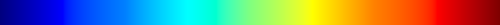# Colormap¶

## Example usage¶

A simple example of applying a colormap on data:

from trollimage.colormap import rdbu
from trollimage.image import Image

img = Image(data, mode="L")

rdbu.set_range(-90 + 273.15, 30 + 273.15)
img.colorize(rdbu)

img.show()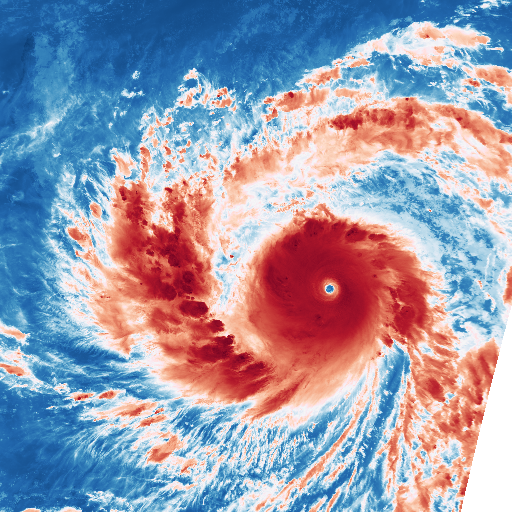A more complex example, with a colormap build from greyscale on one end, and spectral on the other, like this: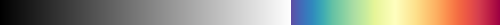from trollimage.colormap import spectral, greys
from trollimage.image import Image

img = Image(data, mode="L")

greys.set_range(-40 + 273.15, 30 + 273.15)
spectral.set_range(-90 + 273.15, -40.00001 + 273.15)
my_cm = spectral + greys
img.colorize(my_cm)

img.show()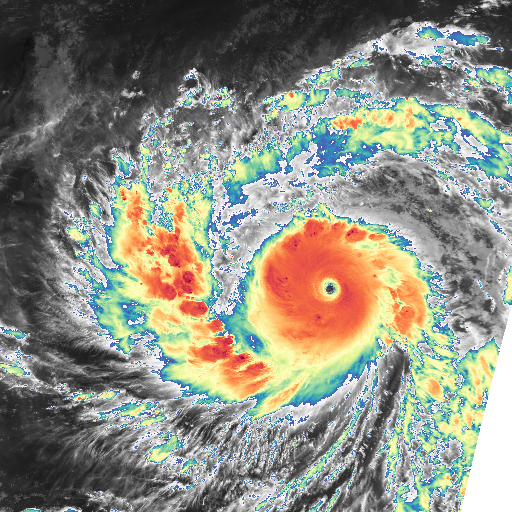Now applying a palette to the data, with sharp edges:

from trollimage.colormap import set3
from trollimage.image import Image

img = Image(data, mode="L")

set3.set_range(-90 + 273.15, 30 + 273.15)
img.palettize(set3)

img.show()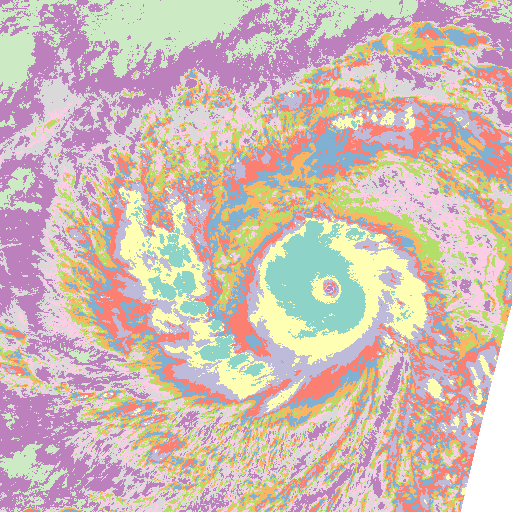## API¶

A simple colormap module.

class trollimage.colormap.Colormap(*tuples, **kwargs)

The colormap object.

Parameters: *args – Series of (value, color) tuples. These positional arguments are only used if the values and colors keyword arguments aren’t provided. values – One dimensional array-like of control points where each corresponding color is applied. Must be the same number of elements as colors and must be monotonic. colors – Two dimensional array-like of RGB or RGBA colors where each color is applied to a specific control point. Must be the same number of colors as control points (values). Colors should be floating point numbers between 0 and 1.

Initialize with tuples of (value, (colors)), like this:

Colormap((-75.0, (1.0, 1.0, 0.0)),
(-40.0001, (0.0, 1.0, 1.0)),
(-40.0, (1, 1, 1)),
(30.0, (0, 0, 0)))


You can also concatenate colormaps together, try:

cm = cm1 + cm2

colorize(data)

Colorize a monochromatic array data, based on the current colormap.

classmethod from_file(filename_or_string: str, colormap_mode: Optional[str] = None, color_scale: numbers.Number = 255)

Create Colormap from a comma-separated or binary file of colormap data.

Parameters: filename_or_string – Filename of a binary or CSV file or a string version of the comma-separate data. colormap_mode – Force the scheme of the colormap data (ex. RGBA). See information below on other possible values and how they are interpreted. By default this is determined based on the number of columns in the data. color_scale – The maximum possible color value in the colormap data provided. For example, if the colors in the provided data were 8-bit unsigned integers this should be 255 (the default). This value will be used to normalize the colors from 0 to 1.

Colormaps can be loaded from .npy, .npz, or comma-separated text files. Numpy (npy/npz) files should be 2D arrays with rows for each color. Comma-separated files should have a row for each color with each column representing a single value/channel. A filename ending with .npy or .npz is read as a numpy file with numpy.load(). All other extensions are read as a comma-separated file. For .npz files the data must be stored as a positional list where the first element represents the colormap to use. See numpy.savez() for more information. The filename should be an absolute path for consistency.

The colormap is interpreted as 1 of 4 different “colormap modes”: RGB, RGBA, VRGB, or VRGBA. The colormap mode can be forced with the colormap_mode keyword argument. If it is not provided then a default will be chosen based on the number of columns in the array (3: RGB, 4: VRGB, 5: VRGBA).

The “V” in the possible colormap modes represents the control value of where that color should be applied. If “V” is not provided in the colormap data it defaults to the row index in the colormap array (0, 1, 2, …) divided by the total number of colors to produce a number between 0 and 1. See the “Set Range” section below for more information. The remaining elements in the colormap array represent the Red (R), Green (G), and Blue (B) color to be mapped to.

See the “Color Scale” section below for more information on the value range of provided numbers.

Color Scale

By default colors are expected to be in a 0-255 range. This can be overridden by specifying color_scale keyword argument. A common alternative to 255 is 1 to specify floating point numbers between 0 and 1. The resulting Colormap uses the normalized color values (0-1).

palettize(data)

Palettize a monochromatic array data based on the current colormap.

reverse(inplace=True)

Reverse the current colormap in place.

Parameters: inplace (bool) – If True (default), modify the colors of this Colormap inplace. If False, return a new instance.
set_range(min_val, max_val, inplace=True)

Set the range of the colormap to [min_val, max_val].

The Colormap’s values will match the range specified even if “min_val” is greater than “max_val”. To flip the order of the colors, use reversed().

Parameters: min_val (float) – New minimum value for the control points in this colormap. max_val (float) – New maximum value for the control points in this colormap. inplace (bool) – If True (default), modify the values inplace. If False, return a new Colormap instance.
to_csv(filename: Optional[str] = None, color_scale: numbers.Number = 255) → Optional[str]

Save Colormap to a comma-separated text file or string.

The CSV data will have 4 to 5 columns for each row where each each row will contain the value (V), red (R), green (B), blue (B), and if configured alpha (A).

The values will remain in whatever range is currently set on the colormap. The colors of the colormap (assumed to be between 0 and 1) will be multiplied by 255 to produce a traditional unsigned 8-bit integer value.

Parameters: filename – The filename of the CSV file to save to. If not provided or None a string is returned with the contents. color_scale – Scale colors by this factor before converting to a CSV. Colors are stored in the Colormap in a 0 to 1 range. Defaults to 255. If not equal to 1 values are converted to integers too.
to_rgb()

Return colormap with RGB colors.

If already RGB then the same instance is returned. If an Alpha channel exists in the colormap, it is dropped.

to_rgba()

Return colormap with RGBA colors.

If already RGBA then the same instance is returned. If not already RGBA, a completely opaque (1.0) color

to_rio()

Convert the colormap to a rasterio colormap.

Note that rasterio requires color tables to have round integer value control points. This method assumes that the range of this Colormap is already in the desired output range and to avoid issues with rasterio will round the values and convert them to unsigned integers.

trollimage.colormap.can_be_block_mapped(data)

Check if the array can be processed in chunks.

trollimage.colormap.colorbar(height, length, colormap)

Return the channels of a colorbar.

trollimage.colormap.colorize(arr, colors, values)

Colorize a monochromatic array arr, based colors given for values.

Interpolation is used. values must be in ascending order.

Parameters: arr (numpy array, numpy masked array, dask array) – data to be colorized. colors (numpy array) – the colors to use (R, G, B) values (numpy array) – the values corresponding to the colors in the array
trollimage.colormap.palettebar(height, length, colormap)

Return the channels of a palettebar.

trollimage.colormap.palettize(arr, colors, values)

Apply colors to data from start values.

Parameters: arr (numpy array, numpy masked array, dask array) – data to be palettized. colors (numpy array) – the colors to use (R, G, B) values (numpy array) – the values corresponding to the colors in the array

## Default Colormaps¶

Colors from www.ColorBrewer.org by Cynthia A. Brewer, Geography, Pennsylvania State University.

### Sequential Colormaps¶

blues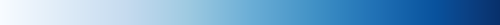greens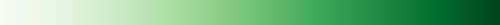greys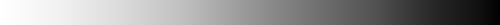oranges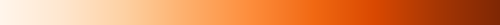purples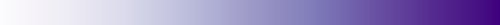reds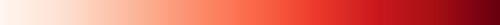bugn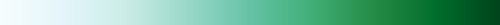bupu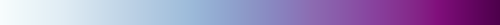gnbu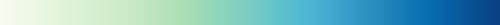orrd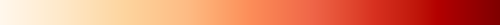pubu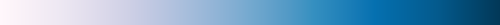pubugn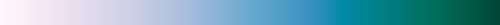purd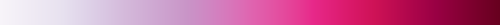rdpu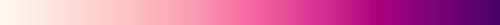ylgn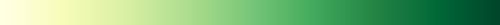ylgnbu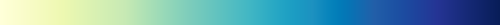ylorbr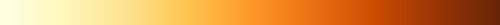ylorrd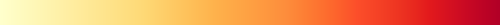### Diverging Colormaps¶

brbg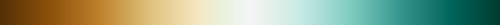piyg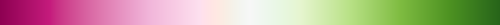prgn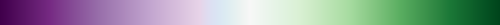puor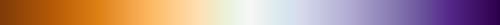rdbu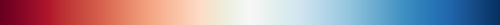rdgy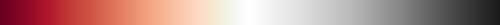rdylbu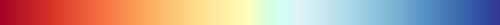rdylgn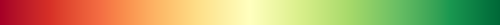spectral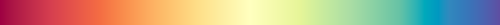### Qualitative Colormaps¶

set1set2set3pairedaccentdark2pastel1pastel2### Rainbow Colormap¶

Don’t use this one ! See here and there why

rainbow